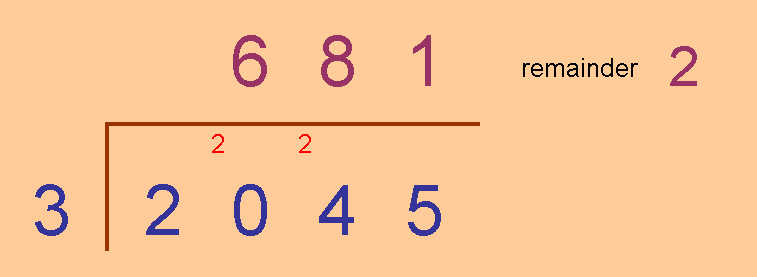# Excel Interactive Resource: Interactive Division

##### Age 11 to 16Challenge Level

This Excel file just lightens the computation burden for division, allowing the teacher and student to discuss the process and explore the relationships between the process elements.Some terms: the Dividend (quantity to be divided) is divided by the Divisor to produce a Quotient with possibly a Remainder.

Personally, I like to have some investigative activity somewhere in the mix, whatever I'm teaching.

Here's a few starter ideas for division (clearly depending on the level of your students):
• You may wish to draw attention to the change in remainder as the dividend increases in steps of 1 or in divisor-size steps.
• For a specific divisor, ask students for a dividend that gives a specific remainder. For example: ask students to choose a dividend between 210 and 220, which when divided by 6, gives a remainder of 3.
• For divisors of 3, and also of 9, invite students to notice a connection between the dividend's digit sum (the sum of the digits in the dividend) and the remainder. And then to account for the relationship observed.